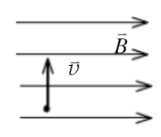$\text{A.}$ 正比于 $B$, 反比于 $v^2$. $\text{B.}$ 反比于 $B$, 正比于 $v^2$. $\text{C.}$ 正比于 $B$, 反比于 $v$. $\text{D.}$ 反比于 $B$, 反比于 $v$.

B